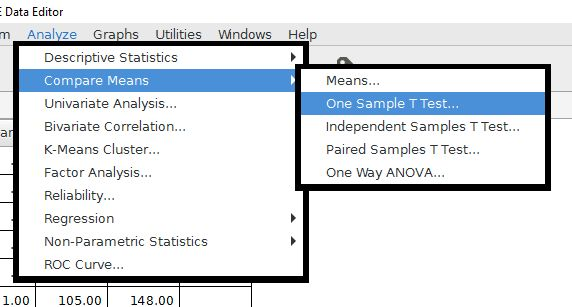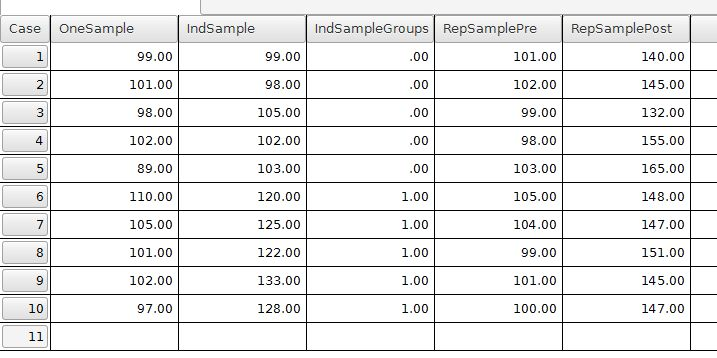# PSPP for Beginners

## The t-test Family

The t-tests are a group of tests that are used to compare two groups or conditions. This test can be used in a wide range of ways. It is often referred to as Student's t-test because it was published anonymously under the name "Student".

Common uses of the t-test include:

• One-sample t-test: For comparing a sample to a population mean
• Independent samples t-test: For comparing two unrelated groups, like an experimental group and a control group
• Repeated-samples t-test (also called paired-samples or dependent-samples t-test): For comparing two related groups

The t tests are located under the Analyze menu in the Compare Means folder. The "Compare means" description is is appropriate because t-tests rely upon comparing group means.We will consider the three forms of the t-test in order, with the example data from the ttestExample.sav file. The data are fictitious data from a standardized math test given to ninth grade high school students. The data represent different research designs that might be used by a teacher to evaluate the effectiveness of a new teaching approach.

The data file looks like this:The variables in this file are set up in the following manner:

• OneSample: Math score data from 10 students.
• IndSample: Math score data from two groups of students involved in an experiment
• IndSampleGroups: Group membership code for the above students
• RepSamplePre: Math scores from 10 students, measured before an experimental intervention
• RepSamplePost: Match scores from 10 students, measured after an experimental intervention